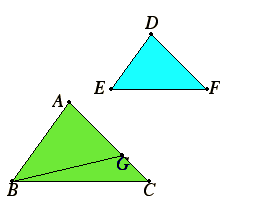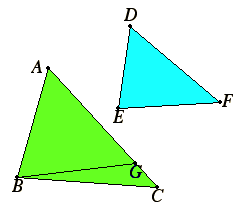# Proposition 7

If two triangles have one angle equal to one angle, the sides about other angles proportional, and the remaining angles either both less or both not less than a right angle, then the triangles are equiangular and have those angles equal the sides about which are proportional.

Let ABC and DEF be two triangles having one angle equal to one angle, the angle BAC equal to the angle EDF, the sides about other angles ABC and DEF proportional, so that AB is to BC as DE is to EF. And, first, each of the remaining angles at C and F less than a right angle.

I say that the triangle ABC is equiangular with the triangle DEF, the angle ABC equals the angle DEF, and the remaining angle, namely the angle at C, equals the remaining angle, the angle at F.If the angle ABC does not equal the angle DEF, then one of them is greater.

I.23

Let the angle ABC be greater. Construct the angle ABG equal to the angle DEF on the straight line AB and at the point B on it.

I.32

Then, since the angle A equals D, and the angle ABG equals the angle DEF, therefore the remaining angle AGB equals the remaining angle DFE.

Therefore the triangle ABG is equiangular with the triangle DEF.

VI.4

Therefore AB is to BG as DE is to EF.

But, by hypothesis, DE is to EF as AB is to BC, therefore AB has the same ratio to each of the straight lines BC and BG. Therefore BC equals BG, so that the angle at C also equals the angle BGC.

I.13

But, by hypothesis, the angle at C is less than a right angle, therefore the angle BGC is also less than a right angle, so that the angle AGB adjacent to it is greater than a right angle.

And it was proved equal to the angle at F, therefore the angle at F is also greater than a right angle. But it is by hypothesis less than a right angle, which is absurd.

Therefore the angle ABC is not unequal to the angle DEF. Therefore it equals it.

I.32

But the angle at A also equals the angle at D, therefore the remaining angle at C equals the remaining angle at F.

Therefore the triangle ABC is equiangular with the triangle DEF.Next let each of the angles at C and F be supposed not less than a right angle.

I say again that, in this case too, the triangle ABC is equiangular with the triangle DEF.

I.5

With the same construction, we can prove similarly that BC equals BG, so that the angle at C also equals the angle BGC.

But the angle at C is not less than a right angle, therefore neither is the angle BGC less than a right angle.

I.17
Thus in the triangle BGC the sum of two angles is not less than two right angles, which is impossible.

Therefore, once more, the angle ABC is not unequal to the angle DEF. Therefore it equals it.

But the angle at A also equals the angle at D, therefore the remaining angle at C equals the remaining angle at F.

Therefore the triangle ABC is equiangular with the triangle DEF.

Therefore, if two triangles have one angle equal to one angle, the sides about other angles proportional, and the remaining angles either both less or both not less than a right angle, then the triangles are equiangular and have those angles equal the sides about which are proportional.

Q.E.D.

## Guide

This is a side-side-angle similarity proposition for triangles. The Elements does not have the analogous side-side-angle congruence proposition for triangles. See the note on congruence theorems after I.26 for more about congruence theorems.